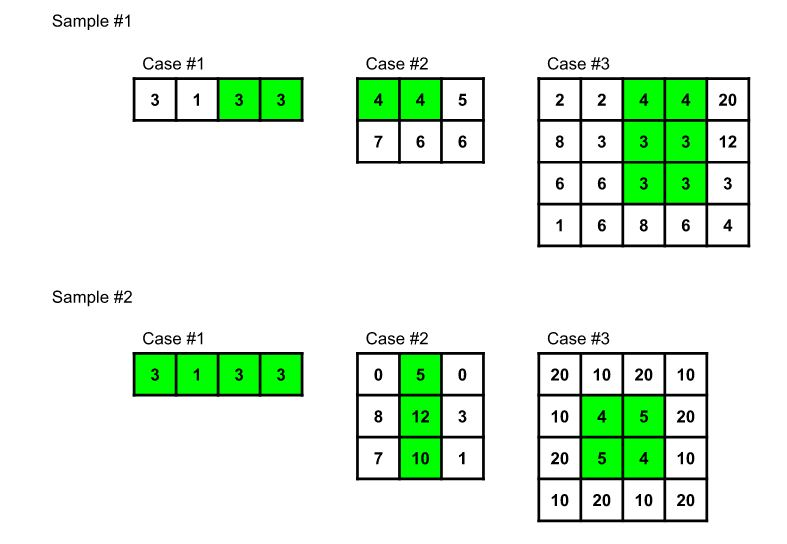## Problem

### Description

Arsh recently found an old rectangular circuit board that he would like to recycle. The circuit board has R rows and C columns of squares.

Each square of the circuit board has a thickness, measured in millimetres. The square in the r-th row and c-th column has thickness Vr,c. A circuit board is good if in each row, the difference between the thickest square and the least thick square is no greater than K.

Since the original circuit board might not be good, Arsh would like to find a good subcircuit board. A subcircuit board can be obtained by choosing an axis-aligned subrectangle from the original board and taking the squares in that subrectangle. Arsh would like your help in finding the number of squares in the largest good subrectangle of his original board.

### Input

The first line of the input gives the number of test cases, T. T test cases follow. Each test case begins with one line containing three integers R, C and K, the number of rows, the number of columns, and the maximum difference in thickness allowed in each row.

Then, there are R more lines containing C integers each. The c-th integer on the r-th line is Vr, c, the thickness of the square in the r-th row and c-th column.

### Output

For each test case, output one line containing Case #x: y, where x is the test case number (starting from 1) and y is the maximum number of squares in a good subrectangle.

### Limits

Time limit: 15 seconds per test set.
Memory limit: 1GB.
1 ≤ T ≤ 50.
1 ≤ R ≤ 300.
1 ≤ C ≤ 300.
0 ≤ Vi, j ≤ 103 for all i, j.

K = 0.

0 ≤ K ≤ 103.

### Sample

Input 1 Output 1
3 1 4 0 3 1 3 3 2 3 0 4 4 5 7 6 6 4 5 0 2 2 4 4 20 8 3 3 3 12 6 6 3 3 3 1 6 8 6 4  Case #1: 2 Case #2: 2 Case #3: 6
Input 2 Output 2
3 1 4 2 3 1 3 3 3 3 2 0 5 0 8 12 3 7 10 1 4 4 8 20 10 20 10 10 4 5 20 20 5 4 10 10 20 10 20  Case #1: 4 Case #2: 3 Case #3: 4

Note: Sample 2 is not valid for Test set 1. Sample 1 will be tested prior to running Test set 1 (the same way samples normally are). However, Sample 2 will not be tested prior to running Test set 2.

The sample cases are illustrated below. For each case, the good subcircuit board with the largest number of squares is highlighted in green.❤采之欲遗谁，所思在远道。❤